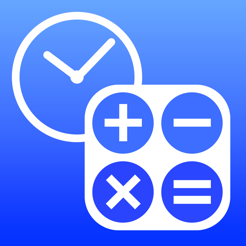## iPhone 截屏

•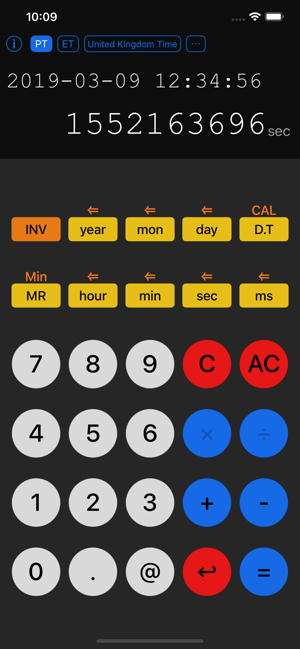•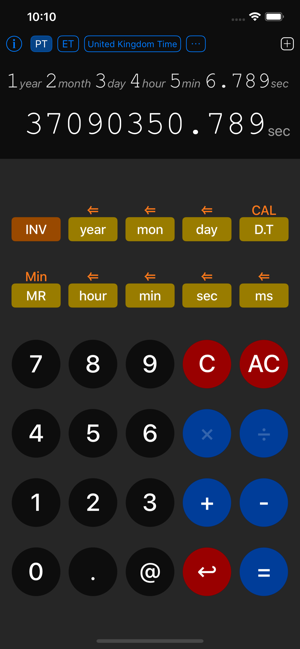•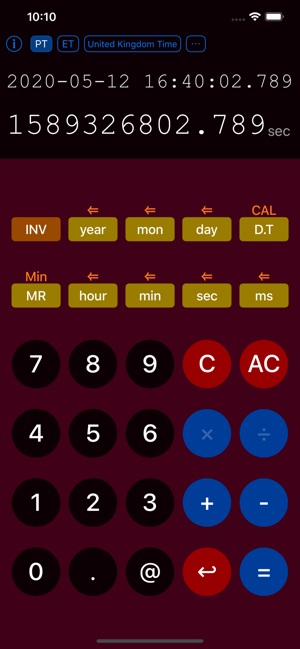•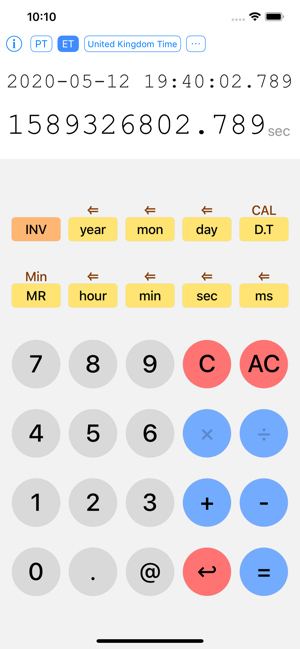## 简介

You can quickly calculate Date / Time Point and Time Interval and unit conversion of time with a pure calculator feeling.

The Date.Time Calculator supports the following calculations.
- Arithmetical operations of numerical values ​​(integer and decimal)
- Arithmetic operations of numerical values ​​and Time Interval
- Operations between Time Interval (addition, subtraction, division)
- Operations of Time Interval and Time Point (addition, subtraction)
- Time Point operation (difference)
- Unit conversion of time
- Interconversion with POSIX Time
- Mutual conversion of time zones

Date.Time Calculator is useful for the following applications.
- What is the date 100 days after today?
- What is the date and time before 32768 seconds from now?
- When it is 1 minute 32.6 seconds per lap, what is the necessary time at 62 laps?
- Furthermore, the time after 62 laps?
- What is the number of days since January 23 this year?
- How many days is 100000 seconds?
- What is the date and time indicated by 1234567890 seconds at POSIX Time?
- What seconds is it now to express with POSIX Time?

Also, it can be utilized variously depending on ingenuity.

## 新内容

Date.Time Calculator 1.3.7 contains improvements and fixes for the following issues
- UI: Improved the layout of the time zone selection area.
- UI: Fix the display font problem of the unit.
- UI: Improved layout and color scheme.

Noriaki HASHIBA

14.9 MB

4+

¥6.00

## 支持

•